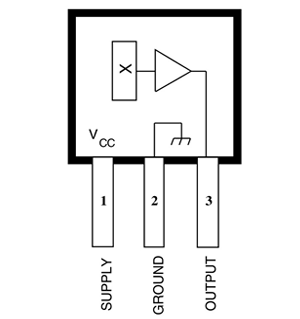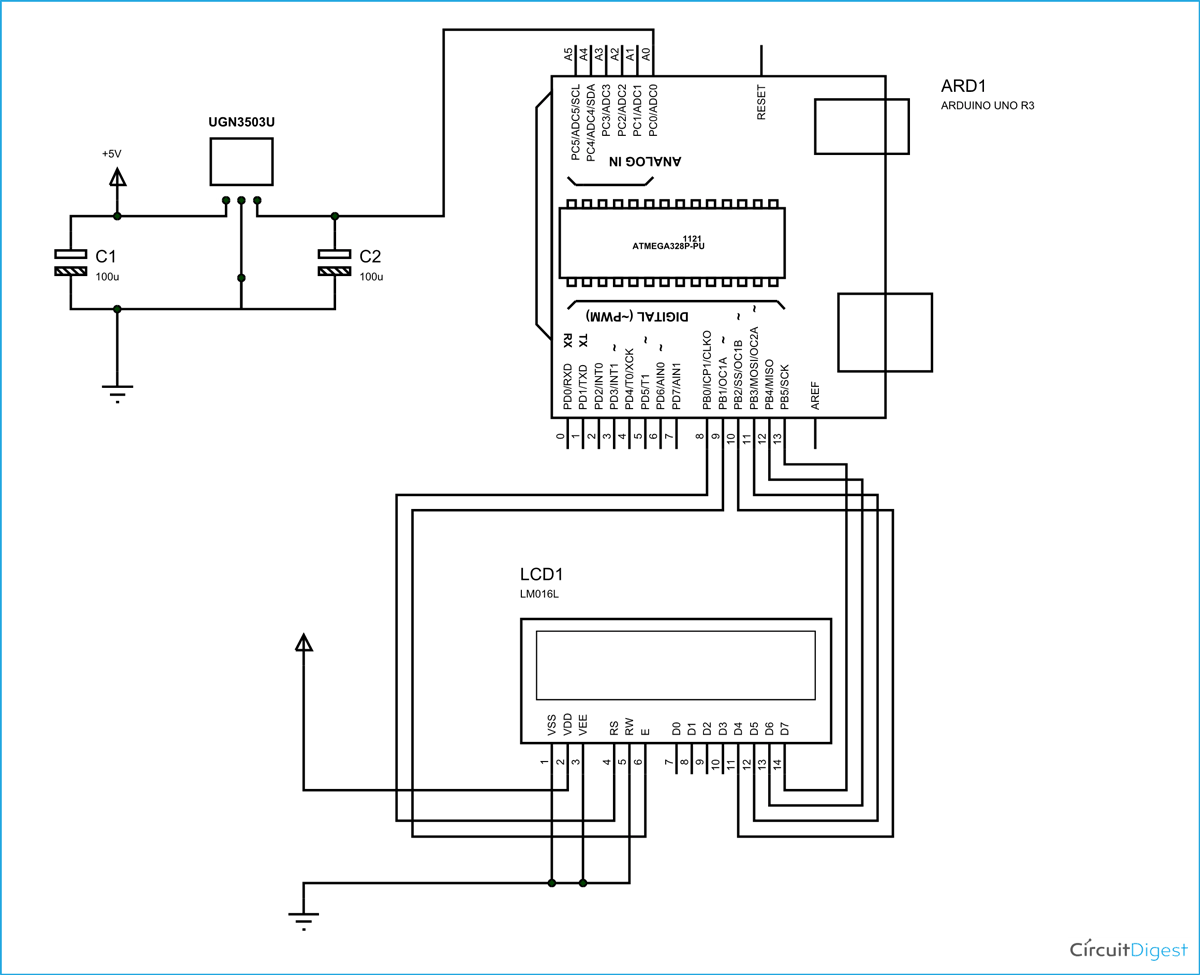In this project we are using the concept of ADC (Analog to Digital Conversion) in ARDUINO UNO. We are going to use a Hall Effect sensor and Arduino uno to measure the field strength of a magnet. The sensor which we have used here is UGN3503U. This is a hall sensor which senses the magnetic field strength and provides a varying voltage at output proportional to the field strength. This sensor picks up field strength in the units of ‘GAUSS’.So with this sensor we will have field strength as varying voltage. By using ADC feature we will convert this voltage to a number. This number represents the field strength and is shown on LCD. [Also check: 16x2 LCD Interfacing with Arduino]

Arduino has six ADC channels. In those any one or all of them can be used as inputs for analog voltage. The UNO ADC is of 10 bit resolution (so the integer values from (0-(2^10) 1023)). This means that it will map input voltages between 0 and 5 volts into integer values between 0 and 1023. So for every (5/1024= 4.9mV) per unit.

In all of this we are going to connect a potentiometer or pot to the ‘A0’ channel, and we are going to show the ADC result in a simple display. The simple displays are 16x1 and 16x2 display units. The 16x1 display unit will have 16 characters and are in one line. The 16x2 will have 32 characters in total 16in 1st line and another 16 in 2nd line. Here one must understand that in each character there are 5x10=50 pixels so to display one character all 50 pixels must work together, but we need not have to worry about that because there a another controller (HD44780) in the display unit which does the job of controlling the pixels (you can see it in LCD unit, it is the black eye at the back).

### Components Required

Hardware: ARDUINO UNO, power supply (5v), JHD_162ALCD (16x2LCD), 100uF capacitor (2pieces), UGn3503U.

Software: arduino IDE (Arduino nightly)

### Circuit Diagram and ExplanationThe above figure shows the circuit diagram for magnetic field measurement using arduino uno.

In 16x2 LCD there are 16 pins over all if there is a back light, if there is no back light there will be 14 pins. One can power or leave the back light pins. Now in the 14 pins there are 8 data pins (7-14 or D0-D7), 2 power supply pins (1&2 or VSS&VDD or GND&+5v), 3rd pin for contrast control (VEE-controls how thick the characters should be shown) and 3 control pins (RS&RW&E).

In the circuit above, you can observe I have only took two control pins, the contrast bit and READ/WRITE are not often used so they can be shorted to ground. This puts LCD in highest contrast and read mode. We just need to control ENABLE and RS pins to send characters and data accordingly.

The connections which are done for LCD are given below:

PIN1 or VSS to ground

PIN2 or VDD or VCC to +5v power

PIN3 or VEE to ground (gives maximum contrast best for a beginner)

PIN4 or RS (Register Selection) to PIN8 of ARDUINO UNO

PIN5 or RW (Read/Write) to ground (puts LCD in read mode eases the communication for user)

PIN6 or E (Enable) to PIN9 of ARDUINO UNO

PIN11 or D4 to PIN10 of ARDUINO UNO

PIN12 or D5 to PIN11 of ARDUINO UNO

PIN13 or D6 to PIN12 of ARDUINO UNO

PIN14 or D7 to PIN13 of ARDUINO UNO

The ARDUINO IDE allows the user to use LCD in 4 bit mode. This type of communication enables the user to decrease the pin usage on ARDUINO, unlike other the ARDUINO need not be programmed separately for using it in 4 it mode because by default the ARDUINO is set up to communicate in 4 bit mode. In the circuit you can see we used 4bit communication (D4-D7). So from mere observation from above table we are connecting 6 pins of LCD to controller in which 4 pins are data pins and 2 pins for control.

### Working

For interfacing an LCD to the ARDUINO UNO, we need to know a few things.

First of all the UNO ADC channels has a default reference value of 5V. This means we can give a maximum input voltage of 5V for ADC conversion at any input channel. Since some sensors provide voltages from 0-2.5V, with a 5V reference we get lesser accuracy, so we have a instruction that enables us to change this reference value.  So for changing the reference value we have (“analogReference();”)

As default we get the maximum board ADC resolution which is 10bits, this resolution can be changed by using instruction (“analogReadResolution(bits);”). This resolution change can come in handy for some cases.

Now if the above conditions are set to default, we can read value from ADC of channel ‘0’ by directly calling function “analogRead(pin);”, here “pin” represents pin where we connected analog signal, in this case it would be “A0”. The value from ADC can be taken into an integer as “int ADCVALUE = analogRead(A0); ”, by this instruction the value after ADC gets stored in the integer “ADCVALUE”.

NOW let’s talk a bit about 16x2 LCD. First we need to enable the header file (‘#include <LiquidCrystal.h>’), this header file has instructions written in it, which enables the user to interface an LCD to UNO in 4 bit mode without any fuzz. With this header file we need not have to send data to LCD bit by bit, this will all be taken care of and we don’t have to write a program for sending data or a command to LCD bit by bit.

Second we need to tell the board which type of LCD we are using here. Since we have so many different types of LCD (like 20x4, 16x2, 16x1 etc.). In here we are going to interface a 16x2 LCD to the UNO so we get ‘lcd.begin(16, 2);’. For 16x1 we get ‘lcd.begin(16, 1);’.

In this instruction we are going to tell the board where we connected the pins, The pins which are connected are to be represented in order as “RS, En, D4, D5, D6, D7”. These pins are to be represented correctly. Since we connected  RS to PIN0 and so on as show in circuit diagram, We represent the pin number to board as “LiquidCrystal lcd(0, 1, 8, 9, 10, 11);”.

After above there all there is left is to send data, the data which needs to be displayed in LCD should be written as “ cd.print("hello, world!");”. With this command the LCD displays ‘hello, world!’. As you can see we need not worry about any this else, we just have to initialize and the UNO will be ready to display data. We don’t have to write a program loop to send the data BYTE by BYTE here.

Once a magnet is brought near the sensor the sensor represents a voltage at output proportional to field, this value is taken up by Uno and shown in LCD. Working of this magnetic field measurement project is further explained through the below C code.

Code

#include <LiquidCrystal.h>

// initialize the library with the numbers of the interface pins

LiquidCrystal lcd(8, 9, 10, 11, 12, 13);/// REGISTER SELECT PIN,ENABLE PIN,D4 PIN,D5 PIN, D6 PIN, D7 PIN

char ADCSHOW;//initializing a character of size 5 for showing the ADC result

void setup()

{

// set up the LCD's number of columns and rows:

lcd.begin(16, 2);

}

void loop()

{

lcd.print("FluxDensity");//showing name

lcd.setCursor(0, 1);//move to second line

lcd.print("(in Gauss):");// showing units

 /* Now since the default reference if 5V and resolution is 10bit so for every 5/1024 = 5mV, we get one increment is count, The sensor provides increment voltage of 1.3V for every 1Gauss increment if field. So we need to divide ADC value by 3.76 for getting the gauss value, now the 0 gauss output of sensor is 2.5V so we need to subtract that first. To hold a 0V read at 0Gauss field. */

// Convert the reading to a char array

lcd.print("G   ");

lcd.setCursor(0, 0);// set the cursor to column 0, line 0

}

Video

Subscribe below to receive most popular news, articles and DIY projects from Circuit Digest

### Related Content

•Karoonjhar
Apr 07, 2016

Hello! I want to know if there's any alternate IC for 'UGN3503U' Please reply.
Thank you.

•Dec 21, 2016

Is there any replacement or similar of UGn3503U ?

•vamsi
Mar 14, 2017

what is alternate for 'UGn3503U'?
else name/what can we ask for UGn3503U

•Turbo
Apr 17, 2017

Alternative to UGN3503 is A1301 from Allegro.
UGN3503 has sensibility 1.3 (typical) mV/Gauss and not 1.3 V/Gauss as written above.
A1301 has exact sensibility as 1.3 mV/Gauss as UGN3503.
If you can't get A1301, you can use A1302 witch has 2.5mV/Gauss.
All sensors UGN3503, A1301 and A1302 you can buy at Aliexpress.

•mike sim
Jul 17, 2017

Hi,
I wish to increase the measuring capability above 900G by using AH49H.
Possible?

If possible and with the existing setup, below is the only changes (code) needed:
AH49H sensitivity is 0.33mV/G
Thus the code had to be change to: repalce 3.76 with 14.8

Not possible cos the supply voltage required is 10V for AH49H.

Thanks

•RACHAEL
Sep 03, 2017

hi ..
pls help me with this error..

it says call overloaded 'String(double)' is ambiuous...

•prashanth
Oct 19, 2017

what all quantites can be measured úsing this project? leaving magnetic field intensity

• AISHA
Oct 21, 2017

The hall effect sensor changes its output based on the magnetic field sourrounding it. So with this ability it can measure the magnetic field intensity. You can also use it to detect the polarity of a magnet. But if you trying to push it further, then there are few places where a hall sensor is used to measure current you can also try those stuff

•Emilio
Oct 31, 2017

Hi! I've got a question, why are you subtracting 515 and how did you got the 3.76 ? Thank you !

•Laura
Nov 13, 2017

does a 3144EUA-S, 3144LUA-S or 44E311 sensor work with this?

•Zeshan Ali
Dec 07, 2017

A3144 can use?

•Anasuha Che Senu
Mar 21, 2018

Hi im interest with this project . Could u help me to get this coding because im used to do this project , the assignment from lecturer. Help me urgent .

•Shami Tiwari
Apr 01, 2018

If we use 3144B7081 sensor than coding will same or different plz help me in that....
Thanks

•Bobba
May 11, 2018

What the capacitor used for and why you choose that value? Does DN6848 Hall Effect sensor can be use as substitute?

• giorgos
May 20, 2019

πως μπορω να δω τους πολους του μαγνητη S-N

• giorgos
May 20, 2019

πως μπορω να δω τους πολους του μαγνητη S-N